An easy-to-implement type of shadow can be created using projection transforms [99,10]. An object is simply projected onto a plane, then rendered as a separate primitive. Computing the shadow involves applying a orthographic or perspective projection matrix to the modelview transform, then rendering the projected object in the desired shadow color.

Here is the sequence needed to render an object that has a shadow cast from a directional light on the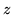axis down onto the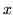,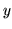plane:

1. Render the scene, including the shadowing object in the usual way.
2. Set the modelview matrix to identity, then call glScalef1.f, 0.f, 1.f(1.f, 0.f, 1.f).
3. Make the rest of the transformation calls necessary to position and orient the shadowing object.
4. Set the OpenGL state necessary to create the correct shadow color.

In the last step, the second time the object is rendered, the transform flattens it into the object's shadow. This simple example can be expanded by applying additional transforms before the glScalef() call to position the shadow onto the appropriate flat object. Applying this shadow is similar to decaling a polygon with another coplanar one. Depth buffering aliasing must be taken into account. To avoid depth aliasing problems, the shadow can be slightly offset from the base polygon using polygon offset, the depth test can be disabled, or the stencil buffer can be used to ensure correct shadow decaling. The best approach is probably depth buffering with polygon offset. This way the depth buffering will minimize the amount of clipping you will have to do to the shadow.

The direction of the light source can be altered by applying a shear transform after the glScalef() call. This technique is not limited to directional light sources. A point source can be represented by adding a perspective transform to the sequence.

Although you can construct an arbitrary shadow from a sequence of transforms, it might be easier to just construct a projection matrix directly. The function below takes an arbitrary plane, defined as a plane equation in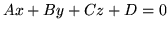form, and a light position in homogeneous coordinates. If the light is directional, the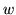value should be 0. The function concatenates the shadow matrix with the current matrix.

static void
{
float  dot;

dot = ground * light +
ground * light +
ground * light +
ground * light;

shadowMat = dot - light * ground;
shadowMat = 0.0 - light * ground;
shadowMat = 0.0 - light * ground;
shadowMat = 0.0 - light * ground;

shadowMat = 0.0 - light * ground;
shadowMat = dot - light * ground;
shadowMat = 0.0 - light * ground;
shadowMat = 0.0 - light * ground;

shadowMat = 0.0 - light * ground;
shadowMat = 0.0 - light * ground;
shadowMat = dot - light * ground;
shadowMat = 0.0 - light * ground;

shadowMat = 0.0 - light * ground;
shadowMat = 0.0 - light * ground;
shadowMat = 0.0 - light * ground;
shadowMat = dot - light * ground;